# Test: Syntax Directed Translation- 2

## 7 Questions MCQ Test GATE Computer Science Engineering(CSE) 2023 Mock Test Series | Test: Syntax Directed Translation- 2

Description
Attempt Test: Syntax Directed Translation- 2 | 7 questions in 21 minutes | Mock test for Computer Science Engineering (CSE) preparation | Free important questions MCQ to study GATE Computer Science Engineering(CSE) 2023 Mock Test Series for Computer Science Engineering (CSE) Exam | Download free PDF with solutions
QUESTION: 1

### Consider the following two statements: P: Every regular grammar is LL(1) Q: Every regular set has a LR(1) grammar Which of the following is TRUE?

Solution:

P:- This is false.
Every regular language is LL(1) meaning we have a LL(1) grammar for it. But we can not say same about every Regular Grammar. For example, every regular language can be represented by Left & Right Linear Grammar, where Left Linear Grammar is not LL(1), Right linear is.

Example aa* we can represent this as S->Sa|a which is not LL(1) ,but S->a|aS is LL(1).

Q:- This is true because of every LL(1) is LR(1).

All regular sets have Right recursive grammar, which is LL(1) & Every LL(1) is LR(1).
We can also say that LR(1) accepts DCFL & Regular languages are subset of DCFL.

QUESTION: 2

### Consider the CFG with {S,A,B) as the non-terminal alphabet, {a,b) as the terminal alphabet, S as the start symbol and the following set of production rules: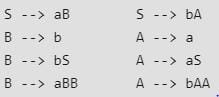Which of the following strings is generated by the grammar?

Solution: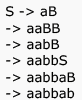QUESTION: 3

### Consider the CFG with {S,A,B) as the non-terminal alphabet, {a,b) as the terminal alphabet, S as the start symbol and the following set of production rules: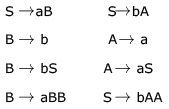For the string aabbab , how many derivation trees are there?

Solution: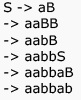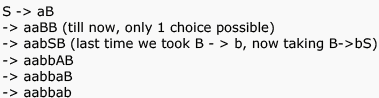QUESTION: 4

Which of the following statements are true?

I. Every left-recursive grammar can be converted to a right-recursive grammar and vice-versa

II. All ∈ -productions can be removed from any context-free grammar by suitable transformations

III. The language generated by a context-free grammar all of whose productions are of the form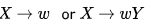(where ω ,  is a string of terminals and  is a non-terminal), is always regular

IV. The derivation trees of strings generated by a context-free grammar in Chomsky Normal Form are always binary trees

Solution:

Statement 1 is true: Using GNF we can convert Left recursive grammar to right recursive and by using reversal of CFG and GNF we can convert right recursive to left recursive.

Statement 2 is false: because if ∊ is in the language then we can't remove ∊ production from Start symbol. (For example L = a*)

Statement 3 is true because right linear grammar generates regular set

Statement 4 is true, only two non-terminals are there in each production in CNF. So it always form a binary tree.

QUESTION: 5

The grammar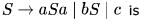Solution:

For LL(1) take First(S). and do intersection between the result. if intersection is Phi then LL(1) else not.

Making a parsing table and checking if there are two or more entries under any terminal. If yes then neither LL(1) nor LR(1).

QUESTION: 6

Consider an ambiguous grammar G and its disambiguated version D. Let the language recognized by the two grammars be denoted by L(G) and L(D) respectively. Which one of the following is true?

Solution:

L (D) = L (G) , Both must represent same language .Also  if we are converting  a grammar from Ambiguous to UnAmbiguous form , we must  ensure that our new new grammar represents the same language as previous grammar.

For ex G1: S->Sa/aS/a; {Ambiguous (2 parse trees for string 'aa')}

G1':S->aS/a;{Unambiguous}

Both represents language  represented by regular expression: a^+

QUESTION: 7

Consider these three grammars.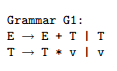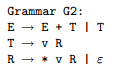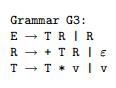Which of the following statements is not true?

Solution:

In G1, T can take the form of v * v * v * v * v ....
In G2, R can take the form * v * v * v ...,  so T can take the form of v * v * v * v ....

Thus, in both G1 and G2, E can take the form v * v * v + v * v * v * v + v * v ....

These are the algebraic expressions involving only addition and multiplication.

In G3, T can take the form v * v * v * v, so R can take the form + v * v + v * v. Thus, through the production E ! T R, E can take the form of any arithmetic expression.

However, E can also take the form of non-arithmetic expressions,

as with E --> R --> + T R --> + v R --> + v + T R ---> + v + v R --> + v + v.

In addition, G3 can generate ε , which G1 and G2 cannot.

Thus, while G1 and G2 generate the same language,

G3 generates a somewhat larger language. Incidentally, G1 demonstrates left-recursion because it contains productions of the form X --> X  α ( in addition to non-problematic productions of the form   X --> β ).

Left recursion can be eliminated by introducing a helper non-terminal R and changing the grammar to read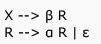Use Code STAYHOME200 and get INR 200 additional OFF Use Coupon Code